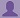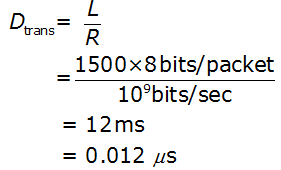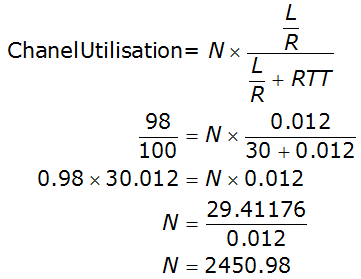SHARE
HELP

To start with, Sr2Jr’s first step is to reduce the expenses related to education. To achieve this goal Sr2Jr organized the textbook’s question and answers. Sr2Jr is community based and need your support to fill the question and answers. The question and answers posted will be available free of cost to all.# Computer Networking : A Top-down Approach

Authors:
James F. Kurose, Keith W. Ross
Exercise:
Problems
Chapter:
Transport Layer
Edition:
6
ISBN:
9780132856201
Question:
15
 Previous Next

###Question

Consider the cross-country example shown in Figure 3.17. How big would the window size have to be for the channel utilization to be greater than 98 percent? Suppose that the size of a packet is 1,500 bytes, including both header fields and data.

###Answer

Consider two systems A and B.

The round-trip propagation delay between A and B (RTT) = 30 ms.

Transmission rate of the link between A and B (R) = 1Gbps = 109bps.

The size of the data packet (L)= 1500 bytes (1500x8 bits)

The following is the formula to calculate the time required to transmit the packet over the 1 Gbps link:where Dtrans is the time required.

Calculate the window size such that the channel utilization is greater than 98%.Therefore, to utilize 98% of the channel, the window size should be 2451 packets approximately.

### Discussions

Post the discussion to improve the above solution.